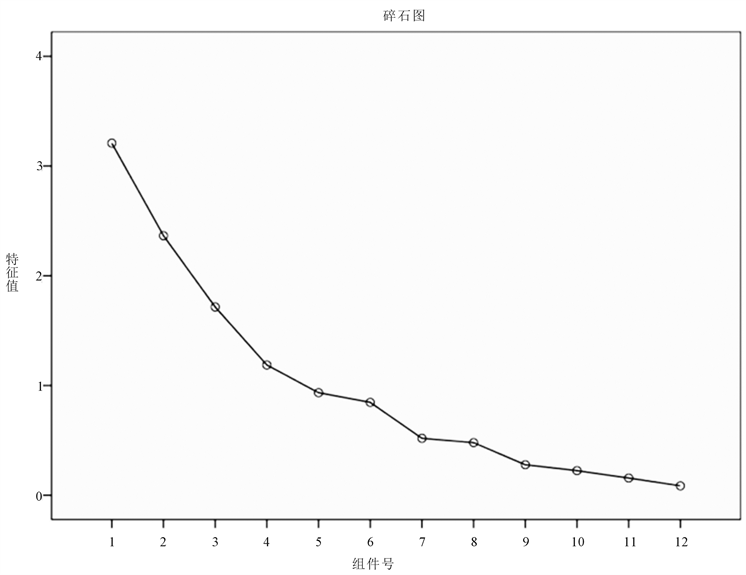# 主成分分析在教学质量分析中的应用The Application of Principal Component Analysis in the Analysis of Teaching Quality

DOI: 10.12677/SA.2018.72031, PDF, HTML, XML, 下载: 817  浏览: 1,326

Abstract: The quality of the teaching of a school is often closely related to the students’ academic performance. In order to study the teaching quality of several primary schools of Shaanxi, we made a principal component analysis of the average scores of 12 subjects in order to know the achievement of each school student . The research results show that the quality of a school’s teaching can be analyzed by student’s academic record. In fact, only a few linear combinations consisting of the average scores of each subject can be considered, which can simplify the problem and improve the efficiency of analysis.

1. 引言

2. 数据收集

3. 主成分分析

3.1. 主成分分析的基本思想

3.2. 主成分分析的数学模型

$X=\left[\begin{array}{cccc}{x}_{11}& {x}_{12}& \cdots & {x}_{1p}\\ {x}_{21}& {x}_{22}& \cdots & {x}_{2p}\\ ⋮& ⋮& \ddots & ⋮\\ {x}_{n1}& {x}_{n2}& \cdots & {x}_{np}\end{array}\right]=\left[{X}_{1},{X}_{2},\cdots ,{X}_{p}\right],\text{}{x}_{j}=\left[\begin{array}{c}{x}_{1j}\\ {x}_{2j}\\ ⋮\\ {x}_{nj}\end{array}\right],j=1,2,\cdots ,p$

$\left\{\begin{array}{l}{F}_{1}={\gamma }_{11}{X}_{1}+{\gamma }_{12}{X}_{2}+\cdots +{\gamma }_{1p}{X}_{p}\\ {F}_{2}={\gamma }_{21}{X}_{1}+{\gamma }_{22}{X}_{2}+\cdots +{\gamma }_{2p}{X}_{p}\\ \text{\hspace{0.17em}}\text{\hspace{0.17em}}\text{\hspace{0.17em}}\text{\hspace{0.17em}}\text{\hspace{0.17em}}\text{\hspace{0.17em}}\text{\hspace{0.17em}}\text{\hspace{0.17em}}\text{\hspace{0.17em}}\text{\hspace{0.17em}}\text{\hspace{0.17em}}\text{\hspace{0.17em}}\text{\hspace{0.17em}}\text{\hspace{0.17em}}\text{\hspace{0.17em}}\text{\hspace{0.17em}}\text{\hspace{0.17em}}\text{\hspace{0.17em}}\text{\hspace{0.17em}}⋮\\ {F}_{p}={\gamma }_{p1}{X}_{1}+{\gamma }_{p2}{X}_{2}+\cdots +{\gamma }_{pp}{X}_{p}\end{array}$

${F}_{j}={\gamma }_{j1}{X}_{1}+{\gamma }_{j2}{X}_{2}+\cdots +{\gamma }_{jp}{X}_{p}$

$Var\left({F}_{j}\right)={\lambda }_{j}$

$Cov\left({F}_{i},{F}_{j}\right)=0$

$F=\gamma X$

$F=\left[\begin{array}{c}{F}_{1}\\ {F}_{2}\\ ⋮\\ {F}_{p}\end{array}\right],\text{\hspace{0.17em}}\gamma =\left({\gamma }_{1},{\gamma }_{2},\cdots ,{\gamma }_{p}\right)$

γ为主成分系数矩阵。

$\frac{\underset{j=1}{\overset{k}{\sum }}{\lambda }_{j}}{\underset{j=1}{\overset{p}{\sum }}{\lambda }_{j}}\ge 85%$

3.3. 主成分分析模型的应用

$\begin{array}{l}{F}_{1}=0.411417{x}_{1}+0.412534{x}_{2}+0.160213{x}_{3}+0.434863{x}_{4}+0.309819{x}_{5}-0.16245{x}_{6}\\ \text{\hspace{0.17em}}\text{\hspace{0.17em}}\text{\hspace{0.17em}}\text{\hspace{0.17em}}\text{\hspace{0.17em}}\text{\hspace{0.17em}}\text{\hspace{0.17em}}-0.30982{x}_{7}-0.18868{x}_{8}+0.334381{x}_{9}-0.24227{x}_{10}-0.12337{x}_{11}+0.080385{x}_{12}\end{array}$

$\begin{array}{l}{F}_{2}=0.235393{x}_{1}+0.313423{x}_{2}+0.05007{x}_{3}+0.25425{x}_{4}+0.103391{x}_{5}-0.45648{x}_{6}\\ \text{\hspace{0.17em}}\text{\hspace{0.17em}}\text{\hspace{0.17em}}\text{\hspace{0.17em}}\text{\hspace{0.17em}}\text{\hspace{0.17em}}\text{\hspace{0.17em}}+0.446076{x}_{7}+0.269206{x}_{8}-0.14761{x}_{9}+0.122248{x}_{10}+0.439573{x}_{11}+0.242546{x}_{12}\end{array}$

$\begin{array}{l}{F}_{3}=-0.07099{x}_{1}+0.140462{x}_{2}+0.415279{x}_{3}+0.000763{x}_{4}-0.31833{x}_{5}-0.247335{x}_{6}\\ \text{\hspace{0.17em}}\text{\hspace{0.17em}}\text{\hspace{0.17em}}\text{\hspace{0.17em}}\text{\hspace{0.17em}}\text{\hspace{0.17em}}\text{\hspace{0.17em}}-0.08779{x}_{7}+0.558795{x}_{8}+0.176341{x}_{9}-0.30459{x}_{10}-0.17787{x}_{11}-0.40536{x}_{12}\end{array}$

$\begin{array}{l}{F}_{4}=0.111978{x}_{1}+0.177146{x}_{2}+0.610374{x}_{3}-0.12483{x}_{4}-0.12391{x}_{5}-0.16246{x}_{6}\\ \text{\hspace{0.17em}}\text{\hspace{0.17em}}\text{\hspace{0.17em}}\text{\hspace{0.17em}}\text{\hspace{0.17em}}\text{\hspace{0.17em}}\text{\hspace{0.17em}}+0.238643{x}_{7}-0.15695{x}_{8}-0.31758{x}_{9}+0.301975{x}_{10}-0.44791{x}_{11}+0.228546{x}_{12}\end{array}$

$\begin{array}{l}{F}_{5}=-0.21108{x}_{1}-0.04242{x}_{2}+0.220397{x}_{3}+0.191425{x}_{4}+0.548406{x}_{5}-0.18004{x}_{6}\\ \text{\hspace{0.17em}}\text{\hspace{0.17em}}\text{\hspace{0.17em}}\text{\hspace{0.17em}}\text{\hspace{0.17em}}\text{\hspace{0.17em}}\text{\hspace{0.17em}}+0.169696{x}_{7}+0.023799{x}_{8}-0.03208{x}_{9}+0.339391{x}_{10}+0.112785{x}_{11}-0.61359{x}_{12}\end{array}$

$\begin{array}{l}{F}_{6}=0.139163{x}_{1}+0.143512{x}_{2}-0.10655{x}_{3}-0.09459{x}_{4}-0.28159{x}_{5}+0.278327{x}_{6}\\ \text{\hspace{0.17em}}\text{\hspace{0.17em}}\text{\hspace{0.17em}}\text{\hspace{0.17em}}\text{\hspace{0.17em}}\text{\hspace{0.17em}}\text{\hspace{0.17em}}-0.06849{x}_{7}-0.19026{x}_{8}+0.541432{x}_{9}+0.646892{x}_{10}-0.05219{x}_{11}-0.16743{x}_{12}\end{array}$Table 1. Interpretation of the total varianceFigure 1. Scree plotTable 3. The coefficient matrix of the principal component

4. 结果分析Table 4. The score of the principal componentFigure 2. A scatter plot of the principal component score

5. 结论与建议

  冷泳林, 王悦. 主成分分析法在学生评教中的应用研究[J]. 信息技术, 2012(12): 11-14.  韩际中, 缪青. 影响农村学校教学质量的客观因素[J]. 黑河教育, 2009(4): 20.  张晓. 主成分分析法在教学评价中的应用[J]. 伊犁师范学院学报, 2009(4): 45-47.  何晓群. 多元统计分析[M]. 第4版. 北京: 中国人民大学出版社, 2015: 113-141.  张尧庭, 方开泰. 多元分析引论[M]. 北京: 科学出版社, 1982.  陈胜可. SPSS统计分析从入门到精通[M]. 第二版. 北京: 清华大学出版社, 2013: 300-315.  Kabacoff, R.I. (2013) R in Action. Manning Publications, 300-315.  何陈. 略谈中小学教育质量评价[J]. 新课程学习: 上, 2015(13): 7.